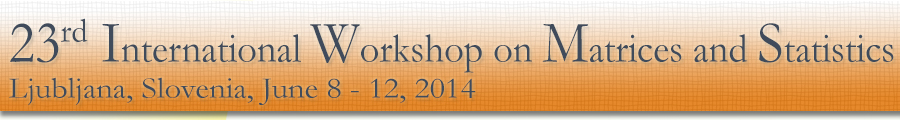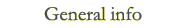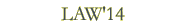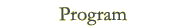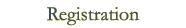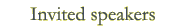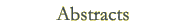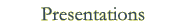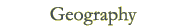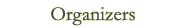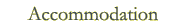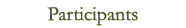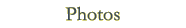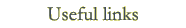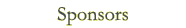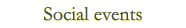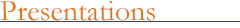Invited ILAS lecture Alexander Guterman: Pólya permanent problem: 100 years after Invited talks Fikri Akdeniz: A new difference-based weighted mixed Liu estimator in partially linear models 1st part 2nd part Tuğrul Dayar: Analyzing Markov chains using Kronecker products Abdelhamid Hassairi: Riesz probability distribution on symmetric matrices and extensions of the Olkin-Rubin characterization Steve Kirkland: Sensitivity analysis for perfect state transfer in quantum walks George P. H. Styan: On 5 x 5 golden magic matrices and 5 x 5 Stifelsche Quadrate Peter Taylor: The role of coupling and the deviation matrix in calculating the value of capacity for queueing systems Yongge Tian: Expansion formulas for inertias of quadratic matrix-valued functions with applications Contributed talks Nesrin Güler: On the BLUEs in two linear models via C. R. Rao's Pandora's box Jeffrey J. Hunter: The computation of the group inverse and related properties of Markov chains via perturbations Lynn R. LaMotte: Using the Gram-Schmidt construction to develop linear models Mika Mattila: Studying the singularity of LCM-type matrices via semilattice structures and their Möbius functions Simo Puntanen: Links between fixed linear model and mixed linear model Burkhard Schaffrin: On a new family of weighted total least-squares algorithms for EIV-models with arbitrary dispersion matrices Aleš Toman: Estimation of the covariance matrix based on two types of the forward search algorithm Julia Volaufova: On R^2 in linear mixed models Hans Joachim Werner: Some results on permutations of matrix products Presentations at Linear Algebra Workshop